# Sample Statistic and Population Parameter Symbols

Author: Sophia Tutorial
##### Description:

Identify a symbol as either a sample statistic or a population parameter.

(more)

Sophia’s self-paced online courses are a great way to save time and money as you earn credits eligible for transfer to many different colleges and universities.*

No credit card required

37 Sophia partners guarantee credit transfer.

299 Institutions have accepted or given pre-approval for credit transfer.

* The American Council on Education's College Credit Recommendation Service (ACE Credit®) has evaluated and recommended college credit for 33 of Sophia’s online courses. Many different colleges and universities consider ACE CREDIT recommendations in determining the applicability to their course and degree programs.

Tutorial
what's covered
This tutorial will explain the distinction between sample statistics and population parameters, with a review of sampling distributions. Our discussion breaks down as follows:

1. Sample Statistics
2. Population Parameters

## 1. Sample Statistics

When you take a sample, it is important to try to obtain values that are accurate and represent the true values for the population. A measure of an attribute of a sample is called a sample statistic

EXAMPLE

In election season, suppose we took a simple random sample of 500 people from a town of 10,000 and found that in this particular poll, 285 of those 500 plan to vote for Candidate Y. That would mean that our best guess for the proportion of the town that will vote for Candidate Y, when the election actually does happen, is 285 out of 500, or 57%. This 57% is a sample statistic.

We don't know what the real proportion is of people who will vote for Candidate Y. We only know that after election day. For now, though, this is our best guess as to the proportion that will vote for Candidate Y. We are using the results of our sample to estimate the value on the population.

In general, the following notations are for the sample statistics that we generate most often. The sample proportion is shown as p-hat. The sample mean is shown as x-bar. Lastly, a sample standard deviation is shown as s.

Statistic
Sample proportion =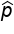Sample Mean =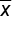Sample Std Dev =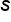hint
Basically, the sample mean is the sum of a certain attribute of a sample divided by the sample size.

terms to know
Sample Statistic
A measure of an attribute of a sample.

Sample Mean
A mean obtained from a sample of a given size. Denoted as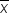.

## 2. Population Parameters

A statistic is a measurement from a sample, and a population parameter is the corresponding measurement for the population. This is something that we can find in a sample. The only way to figure out a parameter is to take a census.

EXAMPLE

In our previous example, the sample proportion was 57%. The population proportion, however, is unknown; we won't know it until election day.

Statistics Parameter
Sample Proportion = 57% Population proportion = ?

A population proportion is denoted as p (without the hat). A population mean is denoted as the Greek letter mu, and a population standard deviation is shown with the Greek letter sigma.

Parameter
Population proportion =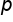Population Mean =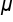Population Std Dev =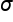hint
Population mean is basically the sum of a certain attribute of a population divided by the population size.

big idea
Parameters are a numerical value that characterizes some aspect of a population.

### IN CONTEXT

The mean GPA of all 2,000 students at a school is 2.9, while the GPA of a sample of 50 students is 3.1

What is the population paramater and the sample statistic?

Based on the information provided, we can identify the following population parameters:

Population size = 2,000
Population mean = μ = 2.9

We can also find the following sample statistics:

Sample size = 50
Sample mean = x̅ = 3.1
terms to know
Population Parameters
Summary values for the population. These are often unknown.
Population Mean
A mean for all values in the population. Denoted as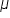.

summary
Statistics are sample measures that we can use to estimate parameters, which are corresponding population measure. It's important to remember that this only works when the sample is carried out well. For instance, if there's bias, then those wouldn't accurately reflect the population measures.

Good luck!

Source: Adapted from Sophia tutorial by Jonathan Osters.

Terms to Know
Population Mean

A mean for all values in the population. Denoted as.

Population Parameters

Summary values for the population. These are often unknown.

Sample Mean

A mean obtained from a sample of a given size.  Denoted as.

Sample Statistics

Summary values obtained from a sample.

Rating